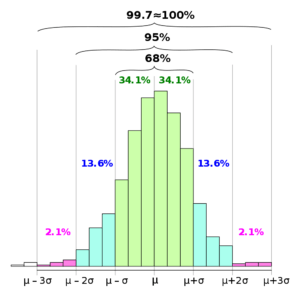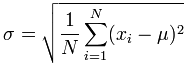# Empirical Rule

Virtually every piece of data will fall within three standard deviations of the mean

## What is the Empirical Rule?

In mathematics, the empirical rule says that in a normal data set, virtually every piece of data will fall within three standard deviations of the mean. The mean is the average of all of the numbers within the set.

The empirical rule is also referred to as the Three Sigma Rule or the 68-95-99.7 Rule because:

• Within the first standard deviation from the mean, 68% of all data rests
• 95% of all the data will fall within two standard deviations
• Nearly all of the data – 99.7% – falls within three standard deviations (the .3% the remains is used to account for outliers, which exist in almost every dataset)### Normal Distribution

The empirical rule came about because the same shape of distribution curves continued to appear over and over to statisticians. The empirical rule denotes a normal distribution. In a normal distribution, all data falls within three standard deviations of the mean. The mean, mode, and median are all equal.

• The mean is the average of all of the numbers in the data set.
• The mode is the number the repeats most frequently within the data set.
• The median is the value of the spread between the highest and lowest numbers within the set.

It means that the mean, mode, and median should all fall at the center of the dataset. Half of the data should be at the higher end of the set, and the other half below.

### Determining the Standard Deviation

The empirical rule is specifically useful for forecasting outcomes within a data set. First, the standard deviation must be calculated. The formula is given below:The complicated formula above breaks down in the following way:

1. Determine the mean of the data set, which is the total of the data set, divided by the quantity of numbers.
2. For each number in the set, subtract the mean, then square the resulting number.
3. Using the squared values, determine the mean for each.
4. Find the square root of the means calculated in step 3.

It is the standard deviation between the three primary percentages of the normal distribution, within which the majority of the data in the set should fall, excluding a minor percentage for outliers.

### Using the Empirical Rule

As mentioned above, the empirical rule is particularly useful for forecasting outcomes within a data set. Statistically, once the standard deviation’s been determined, the data set can easily be subjected to the empirical rule, showing where the pieces of data lie in the distribution.

Forecasting is possible because even without knowing all data specifics, projections can be made as to where data will fall within the set, based on the 68%, 95%, and 99.7% dictates showing where all data should rest.

In most cases, the empirical rule is of primary use to help determine outcomes when not all the data is available. It allows statisticians – or those studying the data – to gain insight into where the data will fall, once all is available. The empirical rules also help to test how normal a data set is. If the data does not adhere to the empirical rule, then it is not a normal distribution and must be calculated accordingly.